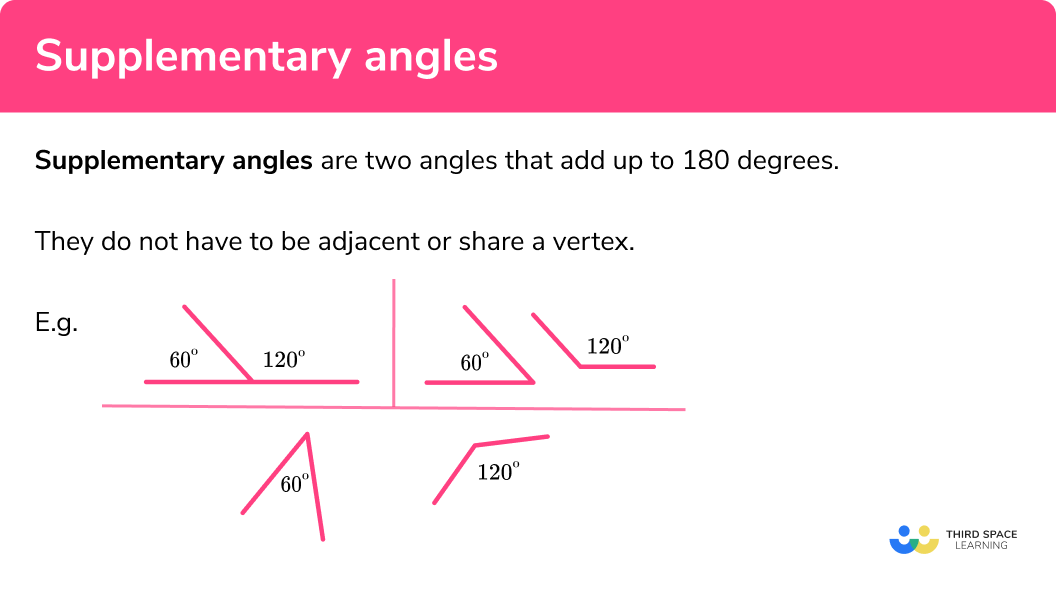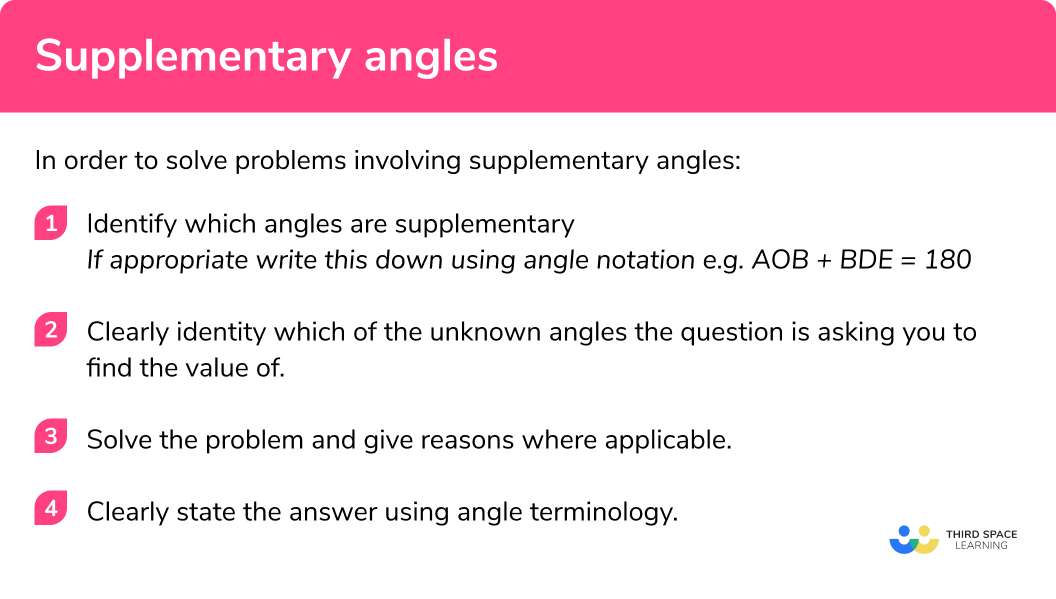# Supplementary Angles

Here we will learn about supplementary angles including how to find missing angles by applying knowledge of supplementary angles to a context.

There are also angles worksheets based on Edexcel, AQA and OCR exam questions, along with further guidance on where to go next if you’re still stuck.

## What are supplementary angles?

Supplementary angles are two angles that add up to 180 degrees. They do not have to be adjacent or share a vertex.

E.g.

When we add together supplementary angles we get a straight line. This is because a straight line is 180 degrees; the same as the total of supplementary angles.

Before we start looking at specific examples it is important we are familiar with some key words, terminology, rules and symbols required for this topic:

• Angle: defined as the amount of turn around a common vertex.
• Vertex: the point created by two line segments (plural is vertices).

• How to label an angle:

We normally label angles in two main ways:

1By giving the angle a ‘name’ which is normally a lowercase letter/symbol such as a, x or y or the greek letter ϴ (theta).

2By referring to the angle as the three letters that define the angle. The middle letter refers to the vertex at which the angle is e.g. see the diagram for the angle we call ABC:

• Angles on a straight line equal 180°:

Angles on one part of a straight line always add up to 180°.

However see the next diagram for an example of where a and b do not equal 180° because they do not meet at one single point on the straight line, i.e. they do not share a vertex and are not adjacent to one another:

• Angles around a point equal 360°:

Angles around a point will always equal 360° See the diagram for an example where angles a, b and c are equal to 360°:

• Vertically opposite angles:

Vertically opposite angles refer to angles that are opposite one another at a specific vertex and are created by two lines crossing. See below for an example:

Here the two angles labelled ‘a’ are equal to one another because they are ‘vertically opposite’ at the same vertex.

The same applies to angles labelled as ‘b’.

Note: Sometimes these are called vertical angles

### What are supplementary angles?## How to solve problems involving supplementary angles

In order to solve problems involving supplementary angles:

1. Identify which angles are supplementary.
If appropriate write this down using angle notation e.g. AOB + BDE = 180
2. Clearly identity which of the unknown angles the question is asking you to find the value of.
3. Solve the problem and give reasons where applicable.
4. Clearly state the answer using angle terminology.

### How to solve problems involving supplementary angles.## Supplementary Angles examples

### Example 1: finding an angle which is supplementary to another

Two angles, x and y, are supplementary and one of them is 17°. What is the size of the other angle?

1. Identify which angles are supplementary.

The two angles are supplementary and therefore equal 180°:

$x+y=180$

2Clearly identity which of the unknown angles the question is asking you to find the value of.

Find the angle that is not 17°

3Solve the problem and give reasons where applicable.

\begin{aligned} x+y&=180 \\ x+17&=180 \hspace{3cm} \text{Replace y with the value 17} \\ x&=163 \hspace{3cm} \text{Subtract 17 from both sides} \end{aligned}

4Clearly state the answer using angle terminology.

The size of the other angle is 163°.

### Example 2: finding an angle which is supplementary to another

Two angles are supplementary. One is double the size of the other. What is the size of the smaller angle?

Identify which angles are supplementary.

Clearly identity which of the unknown angles the question is asking you to find the value of.

Solve the problem and give reasons where applicable.

Clearly state the answer using angle terminology.

### Example 3: finding supplementary angles from a diagram

ABC is a right-angled triangle. Which of the following pairs of angles are supplementary?

Identify which angles are supplementary.

Clearly identity which of the unknown angles the question is asking you to find the value of.

Solve the problem and give reasons where applicable.

Clearly state the answer using angle terminology.

### Example 4: finding a given angle using supplementary angles

Angles A and B are supplementary to one another.

$A = 3x-29$
$B = 3x +17$

Find the size of angle B.

Identify which angles are supplementary.

Clearly identity which of the unknown angles the question is asking you to find the value of.

Solve the problem and give reasons where applicable.

Clearly state the answer using angle terminology.

### Example 5: identifying supplementary angles

AB and CD are parallel. Which pair of angles are supplementary,

HGD and GFB’ or ‘HGD and HGC ’?

Identify which angles are supplementary.

Clearly identity which of the unknown angles the question is asking you to find the value of.

Solve the problem and give reasons where applicable.

Clearly state the answer using angle terminology.

### Example 6: identifying supplementary angles

Which angles in the below trapezium ABCD are supplementary? You must give your answers using correct angle notation.

Identify which angles are supplementary.

Clearly identity which of the unknown angles the question is asking you to find the value of.

Solve the problem and give reasons where applicable.

Clearly state the answer using angle terminology.

### Common misconceptions

These are some of the common misconceptions around the above angle rules

• Incorrectly labelling angles which are supplementary
• Assuming supplementary angles must share a vertex
• Mixing up supplementary angles and complementary angles
• Misuse of the ‘straight line’ rule where angles do not share a vertex
• Finding the incorrect angle due to misunderstanding the terminology

Supplementary angles is part of our series of lessons to support revision on angle rules. You may find it helpful to start with the main angle rules lesson for a summary of what to expect, or use the step by step guides below for further detail on individual topics. Other lessons in this series include:

### Practice supplementary angles questions

1. Two angles ‘ x and y ‘ are supplementary and one of them is 47^{\circ} . What is the size of the other angle?

x=43x=133x=47x=313The two angles are supplementary, so they must have a sum of 180 and 47+133=180 .

2. Two angles ‘ x and y ‘ are supplementary and one of them is 123^{\circ} . What is the size of the other angle?

x=53x=123x=57x=237The two angles are supplementary, so they must have a sum of 180 and 57+123=180 .

3. Two angles are supplementary. One is three times the size of the other. What is the size of the smaller angle?

20^{\circ}45^{\circ}18^{\circ}72^{\circ}The angles make a 180 degree angle. There are three parts in one angle and one part in the other, so four parts in total. If we divide 180 by 4 we get 45 , so this is the size of the smaller angle.

4. Two angles are supplementary. One is 4x – 40 and the other is 5x – 50 . Find the value of x .

x=30x=36x=45x=90The sum of the two angles must equal 180 , so the equation we must solve is 9x-90=180 . Using the standard methods for solving a linear equation gives the solution x=30 .

5. Which angles are supplementary in the diagram below:ACD and DBCABD and DBCDAC and ACDDAB and DBCSince ABD and DBC meet at a point on a straight line, they must be supplementary as angles on a straight line add up to 180 .

6. How many pairs of supplementary angles does a parallelogram have?

 0124By considering angle rules, we know there are four pairs of co-interior angles in a parallelogram. Since co-interior angles add up to 180 they are supplementary.

### Supplementary angles GCSE questions

1. Find the size of the angle marked x .(2 marks)

 180 - 112

(1)

 68^{\circ}

(1)

2.(a) Which two pairs of angles are supplementary in this trapezium?

(3 marks)

(1)

b)  180 - 58

(1)

 122^{\circ}

(1)

3. Work out the size of the smaller angle.(4 marks)

  7x - 4 + x + 16 = 180

(1)

  8x + 12 = 180

(1)

 \begin{aligned}
8x&=168\\
x&=21
\end{aligned} 

(1)

 21 + 16 = 37

(1)

## Learning checklist

You have now learned how to:

• Use conventional terms and notation for angles
• Define angles that are supplementary
• Apply the properties of supplementary angles
• Apply angle facts and properties to solve problems

## Still stuck?

Prepare your KS4 students for maths GCSEs success with Third Space Learning. Weekly online one to one GCSE maths revision lessons delivered by expert maths tutors.

Find out more about our GCSE maths tuition programme.